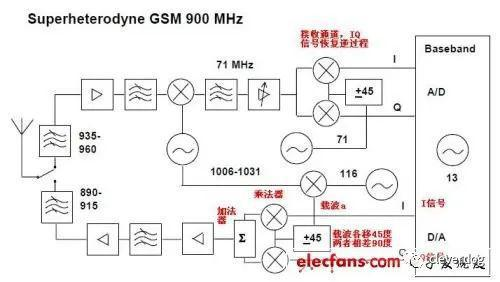# 当前的数字射频芯片，无一例外的用到了I/Q信号，就算是RFID芯片，内部也用到了I/Q信号，然而绝大部分射频人员，对于IQ的了解除了名字之外，基本上一无所知。

I：in-phase  表示同相

cos(a) * cos(b) = 1/2[cos( a + b) - cos(a - b) ]

cos（a－b）＝cos(a)cos(b)＋sin(a)sin(b)

1：数字通讯，单一时间只有一个频点，所以可以移相90度。

2：相加器、相乘器技术很容易实现。接下来就很好办了，大家知道I就是cos(b),Q就是sin(b)

cos(b),   sin(b)

cos(b), -sina(b)

-cos(b),  sin(b)

-cos(b),  -sin(b)

X 提交 下一题